# RBSE Solutions For Class 12 Physics Chapter 14: Atomic Physics | Textbook Important Questions & Answers

The answers are provided for all the questions of Chapter 14 Physics of RBSE Class 12. Students can go through these questions to understand the concepts better and score well in the board examination and entrance examinations for various professional courses. These solutions are provided by the team of experts to provide the best and accurate solutions to the questions. Do check BYJU’S RBSE Class 12 solutions page to get the answers for textbook questions of all subjects.

## Multiple Choice Questions

Q1: The energy of the hydrogen atom in the ground state is -13.6eV. Its energy n=5 level will be

1. -0.5eV
2. -0.85eV
3. -5.4eV
4. -2.7eV

Q2: In the hydrogen atom, if an electron jumps from third to second orbit the wavelength of emitted radiation.

1. 5R/36
2. R/6
3. 36/5R
4. 5/R

Q3: In which of the electromagnetic spectrum Lyman series of hydrogen is found

1. Ultraviolet
2. Infrared
3. Visible
4. X-ray region

Q4: For Lyman series of hydrogen the minimum and maximum wavelengths are

1. 909 Å and 1212 Å
2. 9091 Å and 12120 Å
3. 303 Å and 404 Å
4. 1000 Å and 3000 Å

Q5: The number of spectral lines emitted by hydrogen atom excited to n=4 energy level is

1. 2
2. 3
3. 4
4. 6

## Very Short Answer Type Questions

Q1: Which experiment indicated that the whole positive charge of an atom is confined to a very minute space in the centre?

Answer: Rutherford α particle scattering experiment.

Q2: Write two shortcomings of the Rutherford model regarding the structure of matter.

Answer: Rutherford model regarding the structure of matter failed to explain stability and line spectrum.

Q3: In the hydrogen atom if the angular momentum of an electron has the value h/π in which orbit is it situated?

Then L = h/π

Therefore, the electron is in the second orbit.

Q4: In which region of the electromagnetic spectrum of hydrogen the Lyman series is present?

Answer: Lyman series is present in the ultraviolet region of the electromagnetic spectrum of hydrogen.

Q5: The energy of the electron in the first orbit of a hydrogen-like atom is -27.2eV. What shall be its energy in the third orbit?

En = (-13.6/n2)Z2 eV

n = 1

-27.2 = (-13.6/12)Z2

Z2 = 2

E3 = (-13.6/(3)2)Z2

E3 = -3.02 eV

Q6: What is the ratio of radii of different orbits in a hydrogen atom?

Answer: The ratio is given as 1:4:9…..

Q7: What is the potential energy of an electron in eV in the first orbit of a hydrogen atom?

The potential energy in the first orbit Un = 2En

Where En is the total energy

The value of total energy in the first orbit is En = -13.6 eV

Un = 2(-13.6 )eV

Un = -27.2eV

Q8: If the radius of the first Bohr orbit of a hydrogen atom is 0.5 A, write down the value of the radius of fourth Bohr orbit.

rn = n2r1

r4 = (4)2(0.5)Å

r4 = (16 x 0.5)Å

r4 = 8.0 Å

Q9: Calculate the wavelength of the last line of the Balmer series.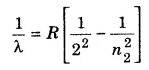n2 = ∞

1/λ = R/4

λ = 4/R

Here R = 1.097 x 107 m-1

λ = (4/1.097)x 10-7 m

λ = 3648 x 10-10 m

λ = 3648 Å

Q10: Write down the formula for quantisation of angular momentum in Bohr’s theory.

Ln = nh/2π (or)

mvnrn = nh/2π

Q11: Write down the name of the series whose few lines fall in the visible region of the hydrogen spectrum.

Q12: On what hypothesis it is possible to explain the second postulate of Bohr theory?

Q1: Write the shortcomings of Thomson’s atomic model.

1. Thomson’s atomic model failed to explain the atom’s stability
2. The theory did not give any information on the nucleus of the atom
3. It did not explain large-angle scattering of particles in Rutherford’s scattering experiment.

Q2: Mention the main consideration in Rutherford’s atomic model.

According to Rutherford’s model

1. The atom has a small and massive central core in which the whole positive charge and mass of the atom is concentrated. This central core is called the nucleus.
2. The nucleus size is much smaller than the size of the atom. The nucleus is surrounded by electrons. The number of negative charges is equal to the positive charges in the nucleus. The atom as a whole is neutral.
3. The electrons revolve around the nucleus like the planets revolving around the sun. The centripetal force required for attraction is provided by the electrostatic attraction between the electrons and the nucleus.

Q3: Explain briefly how the Rutherford atomic model is not able to explain the stability of the atom.

Answer: Rutherford could not explain the stability of an atom because he thought that the electrons revolving in circular orbits will release energy and finally fall into the nucleus.

Q4: Write the shortcomings of Bohr Theory.

1. Using a high-resolution spectrometer it is observed that each line observed earlier was a set of closely spaced lines. Bohr’s model could not explain these fine lines.
2. The Bohr electron did not emit any radiation even though they were in accelerated motion.
3. This theory was valid only for hydrogen and hydrogen-like atoms. It cannot be applied for multi-electron atoms.
4. Bohr’s model did not give information about the intensity of spectral lines.
5. The model failed to explain the splitting of spectral lines due to the electric field and magnetic field.
6. The position and momentum of an electron cannot be determined simultaneously according to Heisenberg’s Uncertainty principle. In Bohr’s model, the momentum and position of an electron are simultaneously discussed.

.

Q5: Hydrogen atom has only one electron but there are many lines in its emission spectrum. Explain briefly how it is possible.

Answer: The hydrogen has only one electron but it has many energy levels. The electron releases a photon when it jumps from higher energy levels to lower energy levels. All these photons appear as lines in its emission spectrum.

Q6: Explain how an element can be identified by studying line spectra?

Answer: The elements release different spectral lines. No two elements will have the same spectral lines.

Q7: In a sample of hydrogen gas most of the atom is in n=1 energy level. When visible light passes through it some spectral lines are absorbed. Lines of which series (Lyman or Balmer) are most absorbed and why?

Answer: The lines of the Lyman series are absorbed since most of the atoms are in (n=1) at a normal state.

Q8: According to Bohr’s theory what is meant by stationary orbit and what is the condition for it?

Answer: Bohr’s orbits are called stationary orbits because he considered that the electrons revolve in these orbits without radiating energy.

The condition for it is that the electrons are permitted to circulate in only those orbits in which the angular momentum of an electron in an integral multiple of 2πh, h is the Planck’s constant.

Q9: Balmer series was observed and analysed before other series. Can you give some reason for it?

Answer: Some of the lines in the Balmer series are found in the visible region. So they are observed and analysed first.

Q10: In the Bohr model the total energy in nth orbit is En and angular momentum is Ln. What is the relation between them?

En ∝ 1/n2 and Ln ∝ n

Therefore En ∝ 1/Ln2

## Essay Type Questions

Q1: Describe briefly the α-particles scattering experiment of Rutherford. How was the nucleus discovered by it?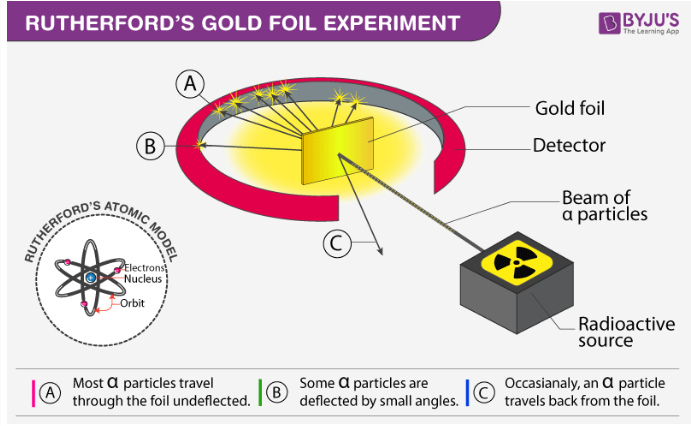Rutherford’s alpha-particle scattering experiment changed the way we think of the atomic structure. Rutherford directed beams of alpha particles from the radioactive Polonium passed through lead bricks to obtain a linear beam. This linear beam is hit on the thin gold foil. In the experiment, Rutherford showed us that the atom was mainly empty space with the nucleus at the centre and electrons revolving around it. When alpha particles were fired towards the gold foil, Rutherford noticed that most of the particles were undeviated or were deviated by a small angle. This led to a conclusion that the atom consisted of an empty space with most of the mass concentrated at the centre in tiny volumes. Hence Thomson’s atomic model, which assumed atoms to be uniformly distributed solid spheres, is not correct.

There were few deflected by 900 or more. It was also observed that few of the particles returned in a direction opposite to the direction of incidence. When an alpha particle comes close to the nucleus without entering into it a large repulsive force acts on it to scatter it by a large angle.

To explain these observations, a new model of the atom was needed. In the new model,

1. All the positive charges of the atom and nearly all the mass are confined to a very small space of 10-15m radius at the centre. This small space is called the nucleus.
2. Most space in an atom is hollow. The positive charge and negative charge in an atom are equal.
3. The electrons revolve around the nucleus in a circular path and the Coulombs attractive force acts as the centripetal force for the circular motion.

Q2: Explain the line spectrum of a hydrogen atom on the basis of Bohr’s atomic model.

According to Bohr’s model, the electrons moved around the nucleus in circular orbits that can have only certain allowed radii. At normal room temperature, all hydrogen nucleus is in the ground state. Atoms will not emit energy in the ground state since there are no states of lower energy level available. When hydrogen gas is given external energy by heat or electric discharge the electrons will transmit from energy levels n=2 to n=3 etc. When these electrons return back to the lower energy level they will emit electromagnetic radiation. According to Bohr’s postulate, the energy emitted when the electron jumps from En2 to En1 is given by

En2 – En1 =hv = hc/λ

1/λ = En2 – En1/hc

The total energy of an electron is given by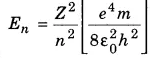Substituting the value of energy to find 1/λ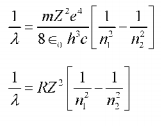Where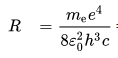, R is called the Rydberg constant

The quantity 1/λ is called the wavenumber.

For hydrogen atom (Z=1) the wavenumber is given as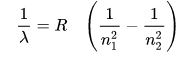Here n1 = 2 and n2 = n, n=3,4,5…

Therefore we get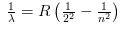The above equation was the same as the equation mentioned by Balmer and Rydberg. This result is a big achievement of Bohr’s theory.

By putting the values of n1 and n2 we get the expression for different series.

n1= 1 and n2 =2,3,4 we get the expression for Lyman series

n1= 2 and n2 =3,4,5 we get the expression for Balmer series

n1= 3 and n2 =4,5,6 we get the expression for Pascher series

n1= 4 and n2 =5,6,7 we get the expression for Bracket series

n1= 5 and n2 =6,7,8 we get the expression for Pfund series